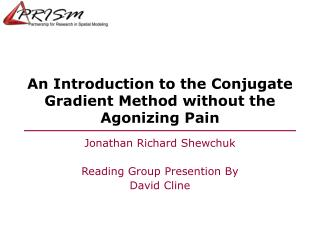DownloadDownload PresentationAn Introduction to the Conjugate Gradient Method without the Agonizing Pain

# An Introduction to the Conjugate Gradient Method without the Agonizing Pain

Télécharger la présentation## An Introduction to the Conjugate Gradient Method without the Agonizing Pain

- - - - - - - - - - - - - - - - - - - - - - - - - - - E N D - - - - - - - - - - - - - - - - - - - - - - - - - - -
##### Presentation Transcript

1. An Introduction to the Conjugate Gradient Method without the Agonizing Pain Jonathan Richard Shewchuk Reading Group Presention By David Cline

2. Linear System Unknown vector (what we want to find) Known vector Square matrix

3. Matrix Multiplication

4. Positive Definite Matrix [ x1 x2 … xn] > 0 * Also, all eigenvalues of the matrix are positive

5. Quadtratic form • An expression of the form

6. Why do we care? The gradient of the quadratic form is our original system if A is symmetric:

7. Visual interpretation

8. Example Problem:

9. Visual representation f(x) f’(x) f(x)

10. Solution • the solution to the system, x, is the global minimum of f. … if A is symmetric, • And since A is positive definite, x is the global minimum of f

11. Definitions • Error • Residual Whenever you read ‘residual’, Think ‘the direction of steepest Descent’.

12. Method of steepest descent • Start with arbitrary point, x(0) • move in direction opposite gradient of f, r(0) • reach minimum in that direction at distance alpha • repeat

13. Steepest descent, graphically

14. Eigen vectors

15. Steepest descent does well: Steepest descent converges in one Iteration if the error term is an Eigenvector. Steepest descent converges in one Iteration if the all the eigenvalues Are equal.

16. Steepest descent does poorly If the error term is a mix of large and small eigenvectors, steepest descent will move back and forth along toward the solution, but take many iterations to converge. The worst case convergence is related to the ratio of the largest and smallest eigenvalues of A, called the “condition number”:

17. Convergence of steepest descent: # iterations “energy norm” at iteration i “energy norm” at iteration 0

18. How can we speed up or guarantee convergence? • Use the eigenvectors as directions. • terminates in n iterations.

19. Method of conjugate directions • Instead of eigenvectors, which are too hard to compute, use directions that are “conjugate” or “A-orthogonal”:

20. Method of conjugate directions

21. How to find conjugate directions? • Gram-Shmidt Conjugation: Start with n linearly independent vectors u0…un-1 • For each vector, subract those parts that are not A-orthogonal to the other processed vectors:

22. Problem • Gram-Schmidt conjugation is slow and we have to store all of the vectors we have created.

23. Conjugate Gradient Method • Apply the method of conjugate directions, but use the residuals for the u values: ui = r(i)

24. How does this help us? • It turns out that the residual ri is A-orthogonal to all of the previous residuals, except ri-1, so we simply make it A-orthogonal to ri-1, and we are set.

25. Simplifying further k=i-1

26. Putting it all together Start with steepest descent Compute distance to bottom Of parabola Slide down to bottom of parabola Compute steepest descent At next location Remove part of vector that Is not A-orthogonal to di

27. Starting and stopping • Start either with a rough estimate of the solution, or the zero vector. • Stop when the norm of the residual is small enough.

28. Benefit over steepest descent

29. Preconditioning

30. Diagonal preconditioning • Just use the diagonal of A as M. A diagonal matrix is easy to invert, but of course it isn’t the best method out there.

31. CG on the normal equations If A is not symmetric, or positive-definite, or not square, we can’t use CG directly to solve However, we can use it to solve the system is always symmetric, positive definite and square. The problem that we solve with this is the least-squares fit but the condition number increases. Also note that we never actually have to form Instead we multiply by AT and then by A.